# Solids

In Geometry, the shape or the figure that has three (even higher) dimensions are known as solids or three-dimensional shapes. The study of the properties, volume and surface area of three-dimensional shapes is called Solid Geometry. Let us go ahead and focus more on the study of geometrical solids.

## Geometric Shapes

The geometrical figures classified based on the dimensions are as follows:

• Zero dimensional shape – A point.
• One dimensional shape – A line that has a length as its dimension.
• Two-dimensional shapes – A figure that has length and breadth as two dimensions. For example – square, triangle, rectangle, parallelogram, trapezoid, rhombus, quadrilateral, polygon, circle etc.
• Three-dimensional shapes – An object with length, breadth and height as three dimensions. For example – cube, cuboid, cone, cylinder, sphere, pyramid, prism etc.
• Higher-dimensional shapes – There are few shapes expressed in dimensions higher than 3, but we usually do not study them in middle-level mathematics.

## What are solids?

In geometry, there are various types of solids. Solids are three-dimensional shapes because they have three dimensions such as length, breadth and height. The bodies which occupy space are called solids.

## Solid or 3D shapes properties

Solids are classified in terms of their properties. To analyze the characteristics and properties of 3-D geometric shapes, count the number of faces, edges, and vertices in various geometric solids. Let us discuss the properties and formulas for the different solid shapes.

 Solid Shape Figure Property Volume Formula (Cubic Units) Surface Area Formula (Square Units) Cube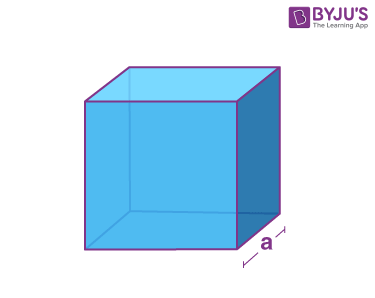Face – square (6) vertices – 8 Edges – 12 a3 6a2 Cuboid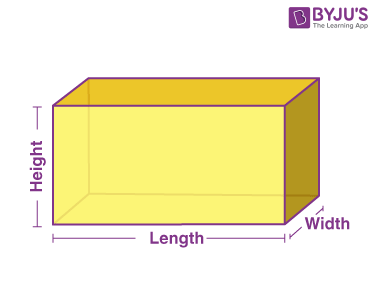Face – Rectangle (6) vertices – 8 Edges – 12 l × b × h 2(lb+lh+hb) Sphere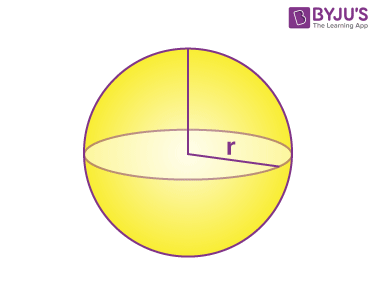Curved surface = 1 Edges = 0 Vertices = 0 (4/3)πr3 4πr2 Cylinder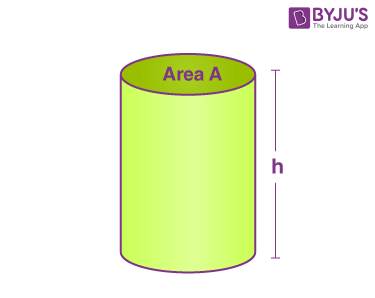Flat Surface = 2 Curved Surface = 1 Face = 3 Edges =2 Vertices =0 πr2h 2πr(r+h) Cone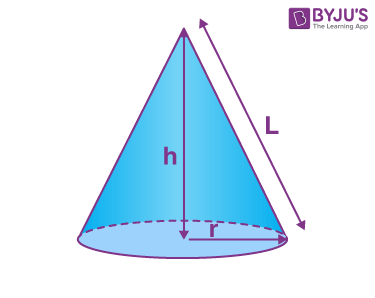Flat Surface = 1 Curved Surface = 1 Face = 2 Edges = 1 Vertices =1 (⅓)πr2h πr(r+l)

### Solids Examples

Question 1:

Find the volume and surface area of a cube whose side is 5 cm.

Solution:

Side, a = 5 cm

The volume of a cube formula is:

The volume of a cube = a3 cubic units

V = 53

V = 5 × 5 × 5

V =125 cm3

Therefore, the volume of a cube is 125 cubic centimeters

The surface area of a cube = 6a2 square units

SA = 6(5)2 cm2

SA = 6(25)

SA = 150 cm2

Therefore, the surface area of a cube is 150 square centimeters

Question 2:

Find the volume of the sphere of radius 7 cm.

Solution:

Given radius of the sphere = r = 7 cm

Volume of sphere = 4/3 πr3

= (4/3) × (22/7) × 7 × 7 × 7

= 4 × 22 × 7 × 7

= 4312 cm3

Question 3:

Find the total surface area of a cuboid of dimensions 8 cm × 5 cm × 7 cm.

Solution:

Given dimensions of a cuboid: 8 cm × 5 cm × 7 cm

That means, length = l = 8 cm

Breadth = b = 5 cm

Height = h = 7 cm

Total surface area of a cuboid = 2(lb + bh + hl)

= 2[8(5) + 5(7) + 7(8)]

= 2(40 + 35 + 56)

= 2 × 131

= 262 cm2

Register with BYJU’S – The Learning App and also download the app to explore engaging videos.

## Frequently Asked Questions (FAQs) on Solids

Q1

### List out the different types of solid shapes.

The different types of solid shapes are:
Cube
Cuboid
Sphere
Cylinder
Cone
Prism
Pyramid
Q2

### What is the Surface Area Formula for sphere and cylinder?

Surface area of Sphere =4πr2

Surface area of Cylinder =2πr(r+h)

Q3

### Find the total surface area of a cuboid of dimensions 5 cm × 6 cm × 7 cm.

Given: Dimensions of a cuboid: 5 cm × 6 cm × 7 cm
Length = l = 5cm, Breadth = b = 6 cm and Height = h = 7 cm
Total surface area of a cuboid = 2(lb + bh + hl)
= 2[5(6) + 6(7) + 7(5)] = 2(30+42+35)
= 2 × 80
= 160 cm2
Q4

### What is solid or 3D?

In Geometry, the shape or the figure that has three dimensions (length, breadth and height), are known as solids or three-dimensional shapes.
Example:-Cube, Cuboid and Sphere
Q5

### Find the volume of the sphere of radius 5 cm.

Given: radius of the sphere = r = 5 cm
Volume of sphere =4/3 π r3
=4/3 × π × 53
=4/3 × π × 125
=523.59 cm3